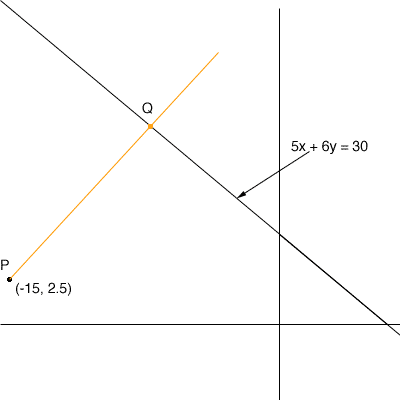Quandaries and Queries Find the shortest distance from the point (-15, 2.5) to the line 5x+6y=30.   The problem I am having is substituting the point into the perpendicular line. I'm not sure how to do it. I really need this answer soon! Please respond as soon as you can. Thanks. carly. Hi Carly,The line 5x + 6y = 30 can be rewritten y = -5/6 x + 5 So its slope is -5/6 and hence a line perpendicular to it has slope 6/5. Hence the line through (-15, 2.5) and perpendicular to the line you were given is (y - 2.5) =  6/5 (x + 15) (I use the point-slope form for the equation of the line but you may use another form.) Simplify the equation and solve the two equations to find the point Q on the diagram. The distance you want is the distance from P to Q. Penny Go to Math Central